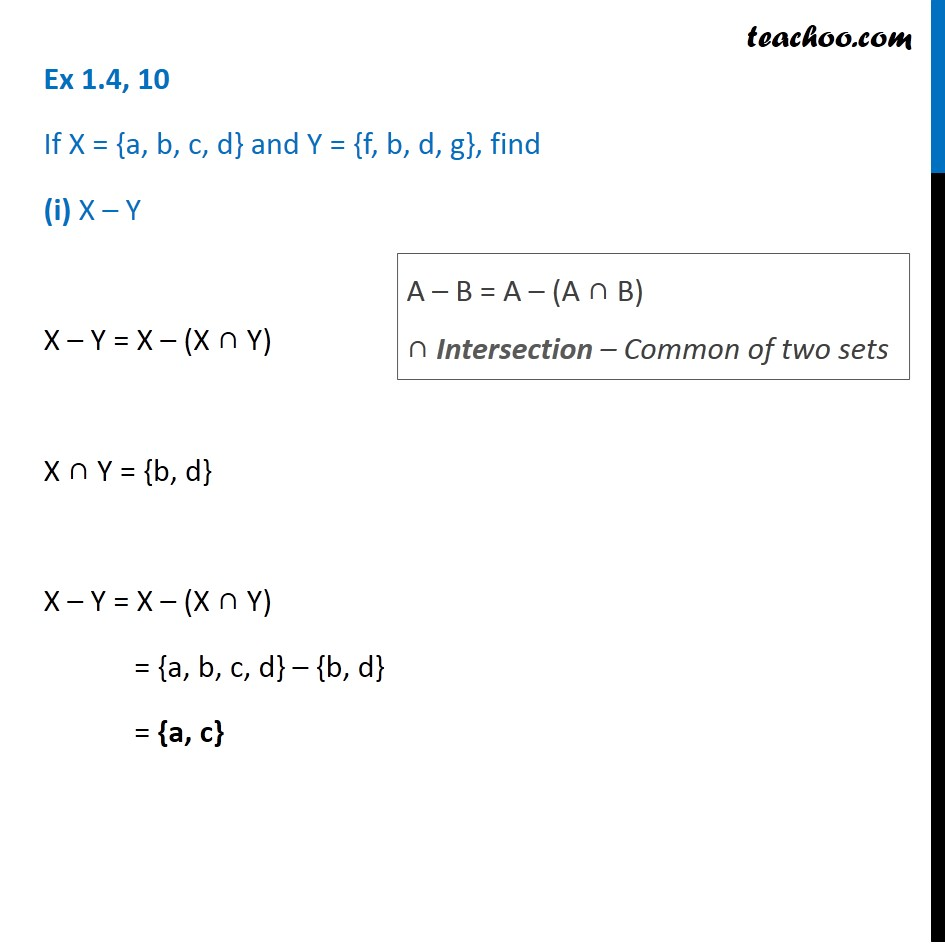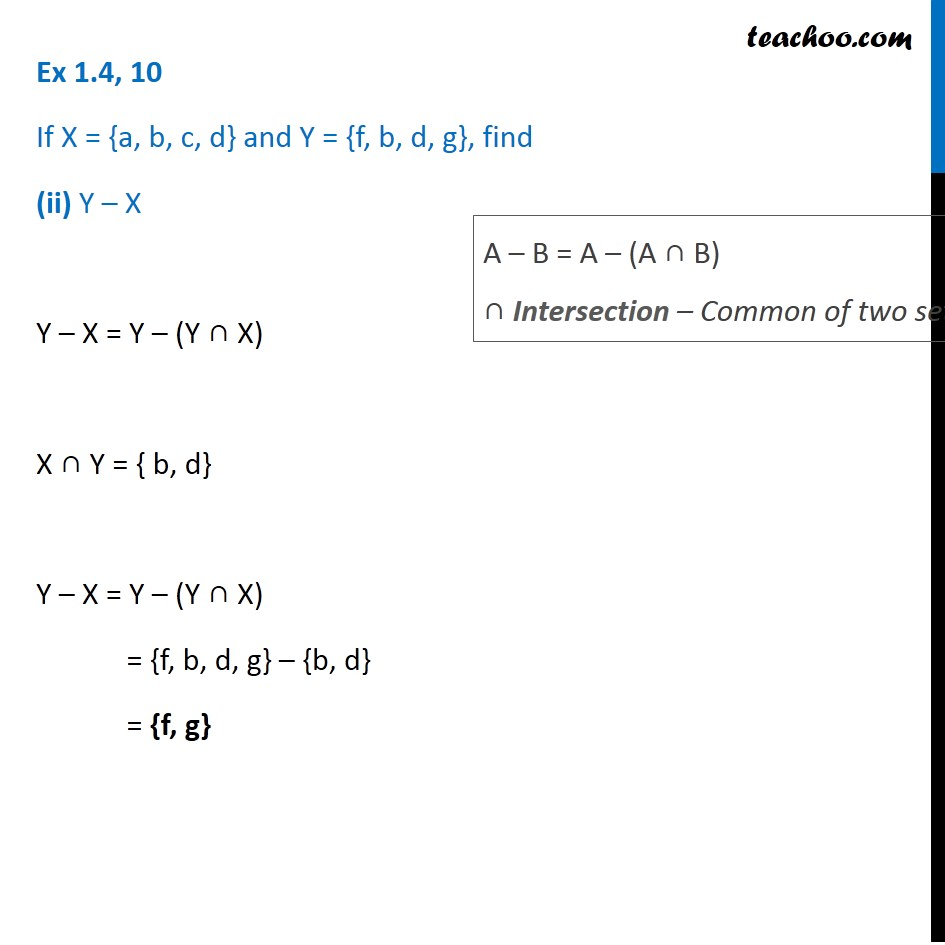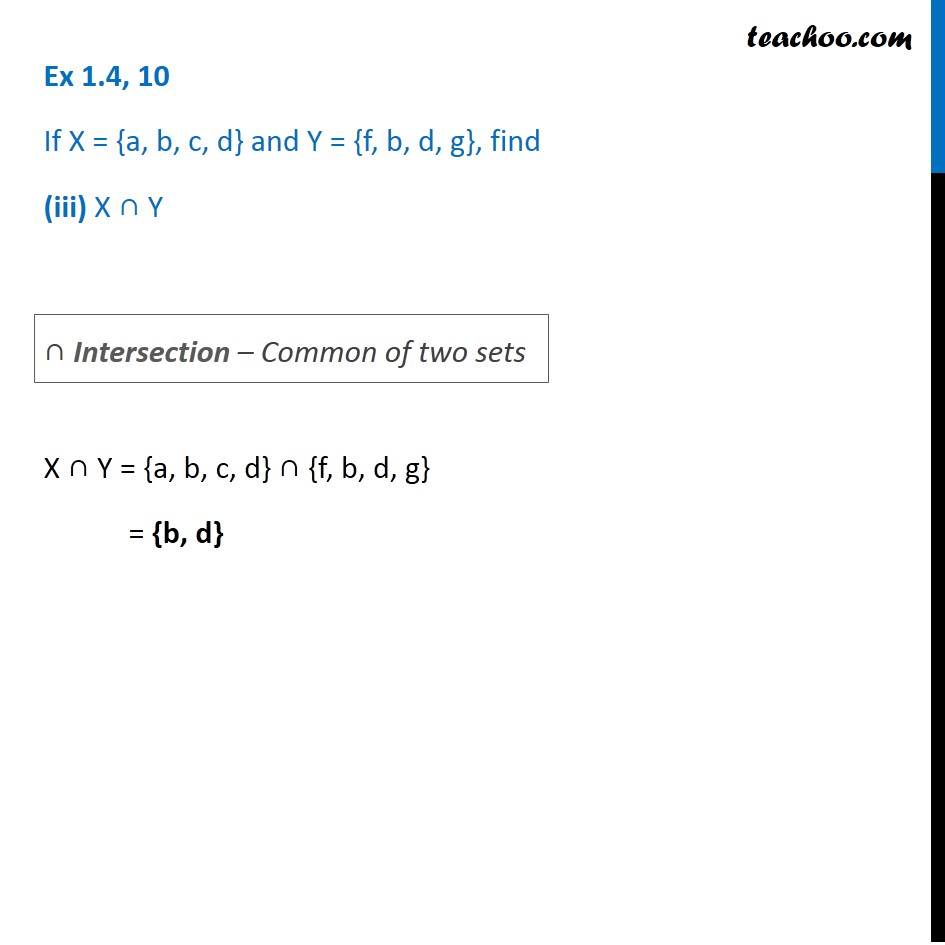1. Chapter 1 Class 11 Sets
2. Concept wise
3. Difference of sets

Transcript

Ex 1.4, 10 If X = {a, b, c, d} and Y = {f, b, d, g}, find (i) X – Y X – Y = X – (X ∩ Y) X ∩ Y = {b, d} X – Y = X – (X ∩ Y) = {a, b, c, d} – {b, d} = {a, c} A – B = A – (A ∩ B) ∩ Intersection – Common of two sets Ex 1.4, 10 If X = {a, b, c, d} and Y = {f, b, d, g}, find (ii) Y – X Y – X = Y – (Y ∩ X) X ∩ Y = { b, d} Y – X = Y – (Y ∩ X) = {f, b, d, g} – {b, d} = {f, g} A – B = A – (A ∩ B) ∩ Intersection – Common of two sets Ex 1.4, 10 If X = {a, b, c, d} and Y = {f, b, d, g}, find (iii) X ∩ Y X ∩ Y = {a, b, c, d} ∩ {f, b, d, g} = {b, d} ∩ Intersection – Common of two sets

Difference of sets